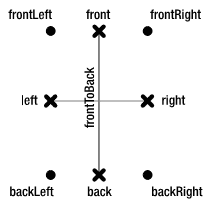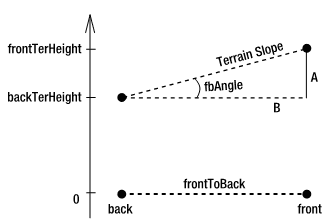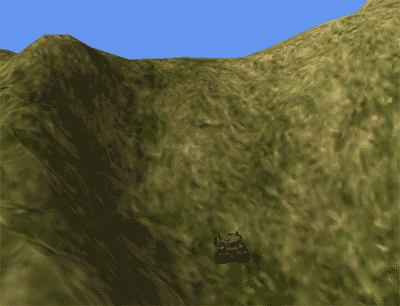# 4.17 根据地形正确倾斜模型

## 解决方案

1. 首先，你想找到模型四个轮胎的最低顶点的位置。
2. 其次，你想获取这四个顶点之下的地形的高度。
3. 下一步，你想获取模型沿Forward和Side向量的旋转以正确地倾斜模型。
4. 最后，你需要找到模型和地形之间的高度差并补偿这个差异。

## 工作原理

### 编写一个自定义模型处理器获取每个ModelMesh最低点的位置

public override ModelContent Process(NodeContent input, ContentProcessorContext context)
{
List<Vector3> lowestVertices = new List<Vector3>();
lowestVertices = FindLowestVectors(input, lowestVertices);

ModelContent usualModel = base.Process(input, context);

int i = 0;
foreach (ModelMeshContent mesh in usualModel.Meshes)
mesh.Tag = lowestVertices[i++];
return usualModel;
}

FindLowestVertices方法遍历模型的所有节点并将每个ModelMesh的最低点位置存储在lowestVertices集合中。有了这个集合，你再将集合中的每个位置存储到对应ModelMesh的Tag属性中。

private List<Vector3>FindLowestVectors(NodeContent node, List<Vector3>lowestVertices)
{
Vector3? lowestPos = null;
MeshContent mesh = node as MeshContent;

foreach (NodeContent child in node.Children)
lowestVertices = FindLowestVectors(child, lowestVertices);
if (mesh != null)
foreach (GeometryContent geo in mesh.Geometry)
foreach (Vector3 vertexPos in geo.Vertices.Positions)
if ((lowestPos == null) || (vertexPos.Y < lowestPos.Value.Y))
lowestPos = vertexPos;
return lowestVertices;
}

### 获取轮子最低顶点的绝对3D坐标

int flID = 5;
int frID = 1;
int blID = 4;
int brID = 0;

Vector3 frontLeftOrig = (Vector3)myModel.Meshes[flID].Tag;
Vector3 frontRightOrig = (Vector3)myModel.Meshes[frID].Tag;
Vector3 backLeftOrig = (Vector3)myModel.Meshes[blID].Tag;
Vector3 backRightOrig = (Vector3)myModel.Meshes[brID].Tag; 

myModel.CopyAbsoluteBoneTransformsTo(modelTransforms);
Matrix frontLeftMatrix = modelTransforms[myModel.Meshes[flID].ParentBone.Index];
Matrix frontRightMatrix = modelTransforms[myModel.Meshes[frID].ParentBone.Index];
Matrix backLeftMatrix = modelTransforms[myModel.Meshes[blID].ParentBone.Index];
Matrix backRightMatrix = modelTransforms[myModel.Meshes[brID].ParentBone.Index];

Vector3 frontLeft = Vector3.Transform(frontLeftOrig, frontLeftMatrix * modelWorld);
Vector3 frontRight = Vector3.Transform(frontRightOrig, frontRightMatrix * modelWorld);
Vector3 backLeft = Vector3.Transform(backLeftOrig, backLeftMatrix * modelWorld);
Vector3 backRight = Vector3.Transform(backRightOrig, backRightMatrix * modelWorld); 

### 获取模型下面的地形的高度Vector3 front = (frontLeft + frontRight) / 2.0f;
Vector3 back = (backLeft + backRight) / 2.0f;
Vector3 backToFront = front - back; 

float frontTerHeight = terrain.GetExactHeightAt(front.X, -front.Z);
float backTerHeight = terrain.GetExactHeightAt(back.X, -back.Z);
float fbTerHeightDiff = frontTerHeight - backTerHeight;### 计算旋转角度

float fbAngle = (float)Math.Atan2(fbTerHeightDiff, backToFront.Length());
Quaternion bfRot = Quaternion.CreateFromAxisAngle(new Vector3(1, 0, 0), -fbAngle);

Vector3 left = (frontLeft + backLeft) / 2.0f;
Vector3 right = (frontRight + backRight) / 2.0f;
Vector3 rightToLeft = left - right;

float leftTerHeight = terrain.GetExactHeightAt(left.X, -left.Z);
float rightTerHeight = terrain.GetExactHeightAt(right.X, -right.Z);
float lrTerHeightDiff = leftTerHeight - rightTerHeight;

float lrAngle = (float)Math.Atan2(lrTerHeightDiff, rightToLeft.Length());
Quaternion lrRot = Quaternion.CreateFromAxisAngle(new Vector3(0, 0, -1), -lrAngle); 

Quaternion combRot = fbRot * lrRot;
Matrix rotatedModelWorld = Matrix.CreateFromQuaternion(combRot) * modelWorld; 

### 将模型放置在正确地高度上

Vector3 rotFrontLeft = Vector3.Transform(frontLeftOrig, frontLeftMatrix * rotatedModelWorld);
Vector3 rotFrontRight=Vector3.Transform(frontRightOrig,frontRightMatrix * rotatedModelWorld);
Vector3 rotBackLeft = Vector3.Transform(backLeftOrig, backLeftMatrix * rotatedModelWorld);
Vector3 rotBackRight= Vector3.Transform(backRightOrig, backRightMatrix * rotatedModelWorld); 

float flTerHeight = terrain.GetExactHeightAt(rotFrontLeft.X, -rotFrontLeft.Z);
float frTerHeight = terrain.GetExactHeightAt(rotFrontRight.X, -rotFrontRight.Z);
float blTerHeight = terrain.GetExactHeightAt(rotBackLeft.X, -rotBackLeft.Z);
float brTerHeight = terrain.GetExactHeightAt(rotBackRight.X, -rotBackRight.Z); 

float flHeightDiff = rotFrontLeft.Y - flTerHeight;
float frHeightDiff = rotFrontRight.Y - frTerHeight;
float blHeightDiff = rotBackLeft.Y - blTerHeight;
float brHeightDiff = rotBackRight.Y - brTerHeight; 

float finalHeightDiff = (blHeightDiff + brHeightDiff + flHeightDiff + frHeightDiff) / 4.0f;
modelWorld = rotatedModelWorld * Matrix.CreateTranslation(new Vector3(0, -finalHeightDiff, 0)); 

### 为动画做准备

myModel.Bones.Transform = originalTransforms;
myModel.Bones.Transform = originalTransforms;
myModel.Bones.Transform = originalTransforms;
myModel.Bones.Transform = originalTransforms;

float time = (float)gameTime.TotalGameTime.TotalMilliseconds / 1000.0f;
Matrix worldMatrix = Matrix.CreateTranslation(new Vector3(10, 0, -12)); // starting position
worldMatrix = Matrix.CreateRotationY(MathHelper.PiOver4*3)*worldMatrix;
worldMatrix = Matrix.CreateTranslation(0, 0, time)*worldMatrix; //move forward
worldMatrix = Matrix.CreateScale(0.001f)*worldMatrix; //scale down a bit
worldMatrix = TiltModelAccordingToTerrain(myModel, worldMatrix, 5, 1, 4, 0);//do tilting magic 

## 代码

namespace ModelVector3Pipeline
{
[ContentProcessor]
public class ModelVector3Processor : ModelProcessor
{
Public override ModelContent Process(NodeContent input, ContentProcessorContext context)
{
List lowestVertices = new List();
lowestVertices = FindLowestVectors(input, lowestVertices);
ModelContent usualModel = base.Process(input, context);

int i = 0;
foreach (ModelMeshContent mesh in usualModel.Meshes)
mesh.Tag = lowestVertices[i++];
return usualModel;
}

private List FindLowestVectors(NodeContent node, List lowestVertices)
{
Vector3? lowestPos = null;
MeshContent mesh = node as MeshContent;

foreach (NodeContent child in node.Children)
lowestVertices = FindLowestVectors(child, lowestVertices);
if (mesh != null)
foreach (GeometryContent geo in mesh.Geometry)
foreach (Vector3 vertexPos in geo.Vertices.Positions)
if ((lowestPos == null) || (vertexPos.Y < lowestPos.Value.Y))
lowestPos = vertexPos;
return lowestVertices;
}
}
} 

private Matrix TiltModelAccordingToTerrain(Model model, Matrix worldMatrix, int flID,
int frID, int blID, int brID)
{
Vector3 frontLeftOrig = (Vector3)model.Meshes[flID].Tag;
Vector3 frontRightOrig = (Vector3)model.Meshes[frID].Tag;
Vector3 backLeftOrig = (Vector3)model.Meshes[blID].Tag;
Vector3 backRightOrig = (Vector3)model.Meshes[brID].Tag;

model.CopyAbsoluteBoneTransformsTo(modelTransforms);
Matrix frontLeftMatrix = modelTransforms[model.Meshes[flID].ParentBone.Index];
Matrix frontRightMatrix = modelTransforms[model.Meshes[frID].ParentBone.Index];
Matrix backLeftMatrix = modelTransforms[model.Meshes[blID].ParentBone.Index];
Matrix backRightMatrix = modelTransforms[model.Meshes[brID].ParentBone.Index];

Vector3 frontLeft = Vector3.Transform(frontLeftOrig, frontLeftMatrix * worldMatrix);
Vector3 frontRight = Vector3.Transform(frontRightOrig, frontRightMatrix * worldMatrix);
Vector3 backLeft = Vector3.Transform(backLeftOrig, backLeftMatrix * worldMatrix);
Vector3 backRight = Vector3.Transform(backRightOrig, backRightMatrix * worldMatrix);

Vector3 front = (frontLeft + frontRight) / 2.0f;
Vector3 back = (backLeft + backRight) / 2.0f;
Vector3 backToFront = front - back;

float frontTerHeight = terrain.GetExactHeightAt(front.X, -front.Z);
float backTerHeight = terrain.GetExactHeightAt(back.X, -back.Z);
float fbTerHeightDiff = frontTerHeight - backTerHeight;
float fbAngle = (float)Math.Atan2(fbTerHeightDiff, backToFront.Length());
Quaternion fbRot = Quaternion.CreateFromAxisAngle(new Vector3(1, 0, 0), -fbAngle);

Vector3 left = (frontLeft + backLeft) / 2.0f;
Vector3 right = (frontRight + backRight) / 2.0f;
Vector3 rightToLeft = left - right;

float leftTerHeight = terrain.GetExactHeightAt(left.X, -left.Z);
float rightTerHeight = terrain.GetExactHeightAt(right.X, -right.Z);
float lrTerHeightDiff = leftTerHeight - rightTerHeight;
float lrAngle = (float)Math.Atan2(lrTerHeightDiff, rightToLeft.Length());
Quaternion lrRot = Quaternion.CreateFromAxisAngle(new Vector3(0, 0, -1), -lrAngle);
Quaternion combRot = fbRot * lrRot;

Matrix rotatedModelWorld = Matrix.CreateFromQuaternion(combRot) * worldMatrix;
Vector3 rotFrontLeft = Vector3.Transform(frontLeftOrig, frontLeftMatrix * rotatedModelWorld);
Vector3 rotFrontRight = Vector3.Transform(frontRightOrig, frontRightMatrix * rotatedModelWorld);
Vector3 rotBackLeft = Vector3.Transform(backLeftOrig, backLeftMatrix * rotatedModelWorld);
Vector3 rotBackRight = Vector3.Transform(backRightOrig, backRightMatrix * rotatedModelWorld);

float flTerHeight = terrain.GetExactHeightAt(rotFrontLeft.X, -rotFrontLeft.Z);
float frTerHeight = terrain.GetExactHeightAt(rotFrontRight.X, -rotFrontRight.Z);
float blTerHeight = terrain.GetExactHeightAt(rotBackLeft.X, -rotBackLeft.Z);
float brTerHeight = terrain.GetExactHeightAt(rotBackRight.X, -rotBackRight.Z);
float flHeightDiff = rotFrontLeft.Y - flTerHeight;
float frHeightDiff = rotFrontRight.Y - frTerHeight;
float blHeightDiff = rotBackLeft.Y - blTerHeight;
float brHeightDiff = rotBackRight.Y - brTerHeight;
float finalHeightDiff = (blHeightDiff + brHeightDiff + flHeightDiff + frHeightDiff) / 4.0f;

worldMatrix = rotatedModelWorld * Matrix.CreateTranslation(new Vector3(0, -finalHeightDiff, 0));

return worldMatrix;
} 

## 扩展阅读2006 - 2023，推荐分辨率1024*768以上，推荐浏览器Chrome、Edge等现代浏览器，截止2021年12月5日的访问次数：1872万9823 站长邮箱